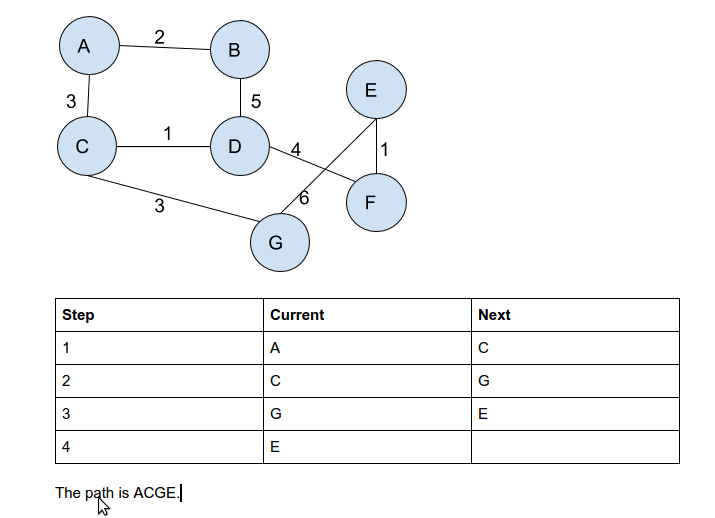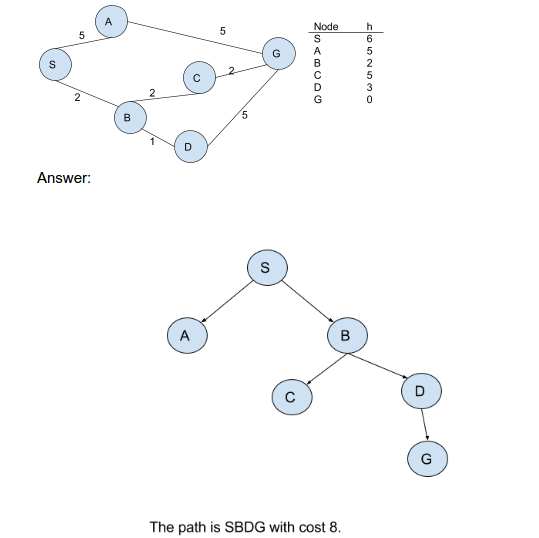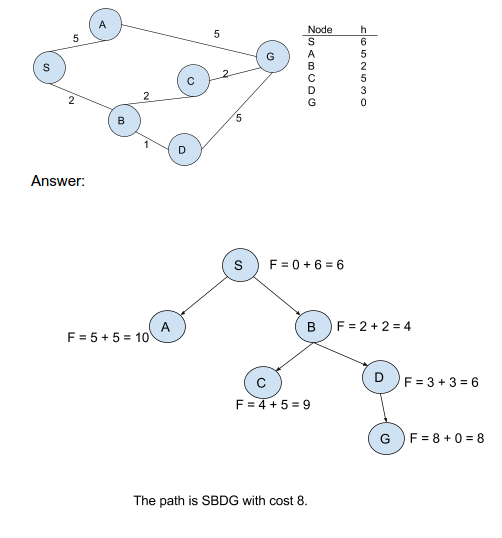### Notes of Artificial Intelligence [CT 653]

#### Search Techniques

Uninformed Search

- They can only expand current state to get a new set of states and distinguish a goal state from non-goal state.
- It is less effective.
- Eg: breadth first search, depth first search depth limit search, etc.

- Proceeds level by level down the search tree.
- Start from root node explores all its children from left to right.
- If no solution is found, expands left most child of root node, then expands second node at depth 1 and so on.
- Eg: find path from A to D.

Properties of BFS
1. Complete if goal node is at finite depth guaranteed to find shortest path
2. Time complexity: O(b^(d+1))
For the worst case with branching farcor b and depth d;
No of nodes generated=1 +b+b^2+…………….+b^(d+1)-b
3. Space complexity: O(b^(d+1))
High time and space requirements.

Depth first search (DFS)

- Proceeds down a single branch of tree at a time.
- Expands root node, then leftmost child of root node,then leftmost child of that node and so on
- Expands node at deepest level

Properties of DFS
1. Incomplete as it may get stuck down going an infinite branch.
2. The first solution found may not be shortest
3. Space complexity: O(b^d)
4. Time complexity:O(b*d)

Depth Limit Search

- Perform depth first search only to a pre-specified depth limit l.
- Incomplete as solution may be beyond l.
- Not optimal
- Time complexity=O(b^l)
- Space complexity=O(b*l)

Informed Search

- They have problem definition along with problem specific knowledge.
- They evaluate which of the nodes on frontier are most promising.
- Improves efficiency
- Develop domain specific heuristic function h(n)
- That guesses the cost of getting to the goal from node n.
- Eg: best first search, hill climbing search etc.

Hill Climbing search

- It is a depth first search that selects the next state based on the estimates of heuristics such that the goodness of next state is higher than goodness of current state.

- Algorithms:
a) Initialize root node to initial state.
b) If root mode is goal, return success and exit.
c) Otherwise, expand current state/
d) Choose the state with highest heuristic
e) If goodness(next)f) Else, current <- next
g) Repeat from step2.

- Problems:
a) Local maxima
b) Plateau
c) Ridges

- Solutions:
a) Backtrack
b) Big jump in some direction
c) Bi directional searchSimulated Annealing

- It is extension of hill climbing that allows downhill step to escape local maxima
- It picks a random move.
- If move improves situation, it is executed
- Else, it moves with some probability less than 1.
- Complete
- Not optimal

- Algorithm
a) Current<-initial state
b) T(control problem of downward step)<-schedule[t]
c) If t=0, return current and stop
d) Next<-random successor of current
e) ΔE<-goodness[next]-goodness[current]
f) If ΔE>0,, current <-next
g) Else, current<-next with probe ΔE/t

Best First Algorithm

- It is the combination of BFS and DFS.

- Algorithms
1. Initialize children list and available list
2. Best node<-root node
3. Check if best node is goal
4. If yes, return success
5. Else, expand best node and list its children in both list
a) Select best node from available list
b) Check if goal
- If yes, success
- If no, expand and list its children

Greedy search

- If tries to get as close as it can to the goal.
- It expands node that is closest to the goal.
- Evaluation function f(n)=h(n)
- Not complete (loop)
- Not optimal
- Time complexity:0(b^m)
- Space complexity : 0(b^m)

- Algorithm
1) Initialize current <- initial state
2) Is h(current )=0?
3) If yes , return success
4) If no;
a) Expand the node
b) Current <- node with least f(n) or h(n)
5) Repeat from 2.A* Search

- It combine path cost and heuristic to form evaluation function
- F(n)=g(n)+h(n)
- Complete if h(n) is admissible -> optimal if h(n) is unsistent
- Complexity : O(b^d)Minimax algorithm

- If 2 players take turn to move, it can solve the problem given sufficient resources assuming each player takes best move in each step.
- Maximize your position while minimizing opponent’s
- Utility function measures how good a position I
- It helps to choose next move in n-player game
- Depth limited search
- Complete if finite depth
- Optimal against an optimal opponent
- Time complexity :O(b^m)
- Space complexity : O(b*m)

- Algorithm
1) Generate game tree completely
2) Determine utility of each terminal state
3) Propagate utility values upward in tree by applying Min and Max operators on the nodes in current level
4) At root node use minimum decision to select move with many utility value.

Alpha Beta Pruning

- It prunes away branches that can not possibly influence the final decision
- Time complexity :O(b^(m/2))
- Minimax algorithm is modified with two values α and β
- α:min.score that max is assured of
- β: max.score that min I s assured of

Searching and Evaluation of Search Strategy

-> searching is the process of finding the required states which is performed through state space by constructing a search tree
=> most of the AI problems do not here a simple algorithmic solution , mostly when the sequence of actions required for solution is unknown . in such case, searching provides a systematic trial and error exploration of alternative solutions so as to prove the best possible solution for the given problem. Hence, searching is important in A I
=> the steps involved in searching process are :=
1) Check if the current state is the goal state.
2) if not, expand it to generate new sets of states.
3) CHOOSE ONE OF THE NEW STATES FOR SEARCH.
4) REPEAT 1 TO 3 until goal state is reached or there are no more states to be expanded
=> Search problem s formulated in forms of stares, operations and goals. State describes the world operator is an action that switch one state to another. The operators which are valid for a state is called applicable operator of that state . goal is the state that leads to solution.

Evaluation of search strategy

A search strategy can be evaluated along the following dimensions:=
a) Completeness: does it leads to a solution if exists.
b) Optimality :does it finds least cost solution ?;
c) Time complexity : how long does it take to find a solution
d) Space complexity : how much memory does I need to perform the search
e) Time and space complexity are measured in terms of :
b=> max. branching factor of search tree
d=> depth of least-close solution
m=> max depth of state space.